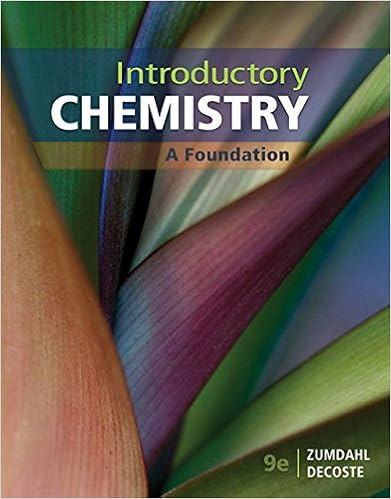# Titanium a strong lightweight corrosion-resistant metal is...

• Test Prep
• coolmanbam258
• 6

This preview shows page 1 - 3 out of 6 pages.

##### We have textbook solutions for you!
The document you are viewing contains questions related to this textbook.The document you are viewing contains questions related to this textbook.
Chapter 10 / Exercise 14
Introductory Chemistry: A Foundation
ZumdahlExpert Verified
CHMY 171 PRACTICE EXAM IV ANSWER KEY Watch your significant figures and units throughout the exam! NAME _____________________ 1. Titanium a strong lightweight corrosion-resistant metal is prepared by the reduction of titanium(IV)chloride with molten magnesium. TiCl 4 + 2Mg Ti + 2MgCl Part. A. (6 pt) Calculate the theoretical yield of Ti if 148kg of TiCl 4 reacts with 46.5 kg of Mg. The theoretical yield is the maximum amount of Ti that can be made. Unless specified, it could be reported in units of moles or grams. I will solve for the theoretical yield in grams. At this point, we don’t know which reactant, the TiCl 4 or the Mg is the limiting reagent, so we must determine how much Ti could be made from the starting amount of TiCl 4 and then separately determine the amount of Ti that could be made from the starting amount of Mg. The reactant that allows us to make less product is the limiting reagent, and the maximum amount of product that could be made if all of the limiting reagent were converted to product is the theoretical yield. 2
Part B. (6 pt) Calculate the % yield if 24345 g of Ti was isolated from the reaction. Again, a percentage is what we got divided by the total possible times 100.
2. (6 pt) What is the mass percent oxygen in Na 2 CO 3
3. (3 pt) Label the following solutions as strongly basic, weakly basic, strongly acidic, or weakly
##### We have textbook solutions for you!
The document you are viewing contains questions related to this textbook.The document you are viewing contains questions related to this textbook.
Chapter 10 / Exercise 14
Introductory Chemistry: A Foundation
ZumdahlExpert Verified
•••